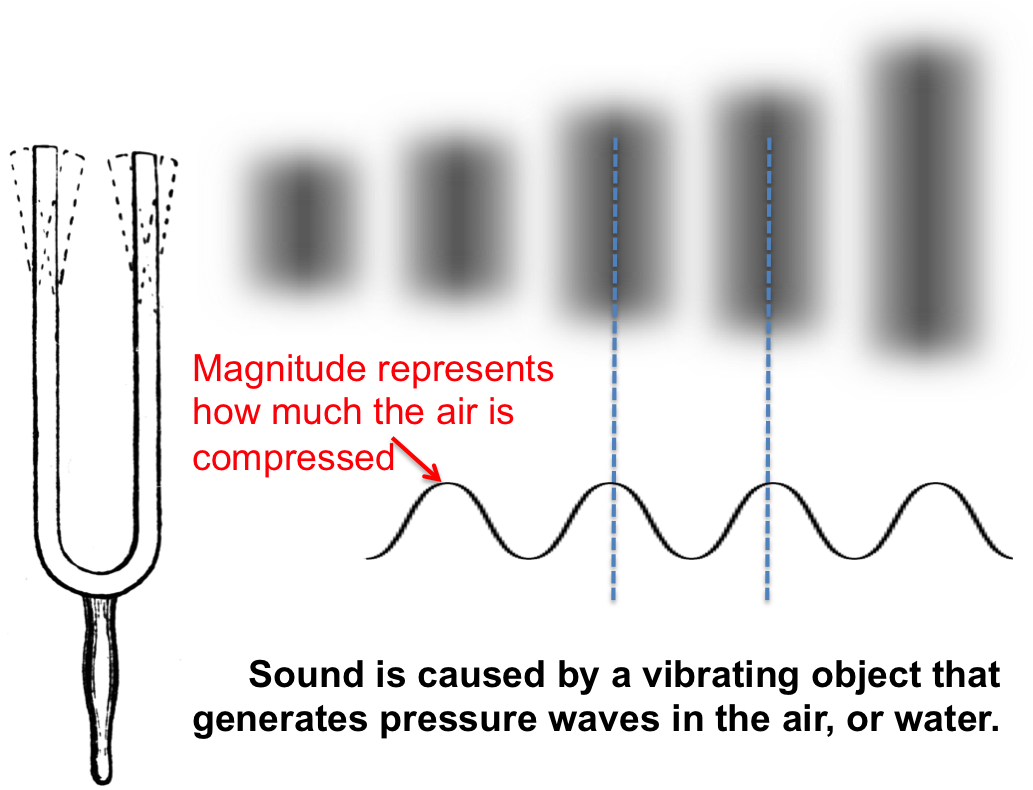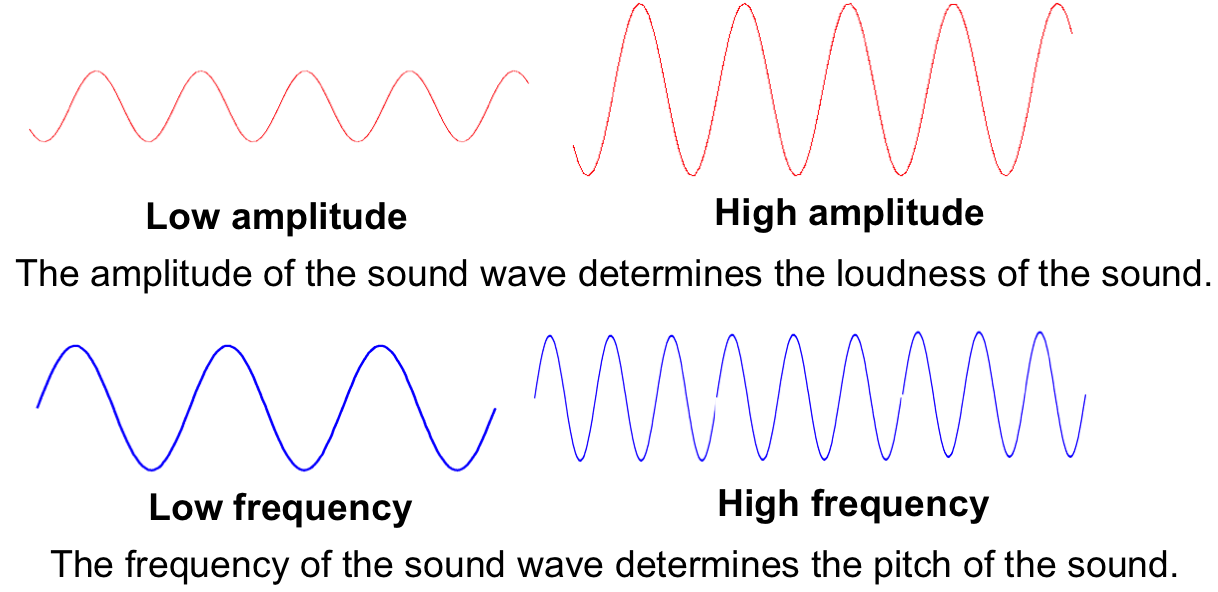Physics-What is sound?

In physics, sound is a mechanical wave of pressure and displacement (vibration) that propagates through a medium, such as air, water or a solid. It may vibrate at any frequency, but humans can only hear sound waves with frequencies between about 20 Hz and 20 kHz. Sound above 20 kHz is called ultrasound and below 20 Hz infrasound.The sound waves are generated by a source, such as a tuning fork, the vibrating diaphragm of a speaker, or the vocal cords of a human being. The vibration of the source pushes the surrounding medium, e.g. air, and creates vibrations in it as well. As the source continues to vibrate, the vibration propagates away from the source at the speed of sound, thus forming the sound wave. At a certain distance from the source, the pressure and particles displacement of the medium vary in time. At any given moment, the pressure and particles displacement of the medium vary in space. Note that the particles of the medium do not propagate with the sound wave. This is intuitively obvious for a solid, and the same is true for liquids and gases (that is, the particles in the medium vibrate, but their average positions over time do not change). During propagation, sound waves can be reflected (echo from a wall), refracted, or attenuated (fading of sound) by the medium.The loudness of the sound is determined by its amplitude, i.e. how much the medium is compressed or decompressed, while the frequency determines the pitch of the sound. The speed of sound depends on the medium that it travels through, and is a fundamental property of the material. The speed of sound is proportional to the square root of the ratio of the bulk modulus of the medium to its density, ν=√K/ρ, which is known as the Newton-Laplace equation. In this equation, K = elastic bulk modulus, ν= velocity of sound, and ρ = density of the material.

Since the physical properties of a material vary with conditions, so does the speed of sound. For example, the speed of sound in gases depends on temperature. In 20 °C(68 °F) air at sea level, it is approximately 343 m/s (1,230 km/h; 767 mph). In fresh water, also at 20 °C, it is approximately 1,482 m/s (5,335 km/h; 3,315 mph). In steel, the speed of sound is about 5,960 m/s (21,460 km/h; 13,330 mph).

Posted Under
Tagged